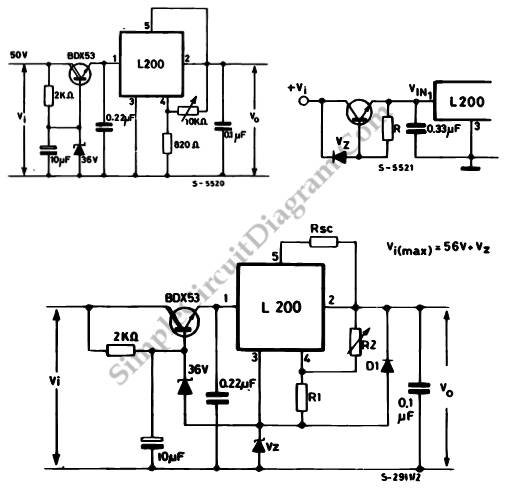# Higher Input Or Output for L200 Voltage Regulator

This is higher input or output for L200 voltage regulator circuit. This circuit is designed to solve the problem that occurs when the input or output voltages are higher than the device can produce. This circuit uses the external components. Here is the circuit :The transistor is used to absorb the excess voltage when there are high input voltage. The SOA of the preregulation transistor and the dissipated power should be calculated. The maximum input volage that can be reached if we use BDX53 is 56 V, so the Vce on transistor is 20V with load current o 2A the operation point remains inside SOA. The ripple at the input of the circuit is reduced by preregulation, so it can recieve an output voltage with negligible ripple.

When we need a high output voltage, we can use a second zener, Vz, that is used to refer an IC’s ground pin to a potential other than zero. To protect the output from short circuit, this circuit uses diode D1. [Source: STMicroelectronics Application Note]